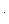## Adding (and Subtracting) Fractions Tips

The “standard” way to add (or subtract) fractions is to put them over a common denominator first. So, the fraction problemis## Another Way To Teach Fraction Division

The classic way to teach fraction division is like this. To work out, say,we ‘turn the second fraction upside-down’, to getand then the division question becomes a multiplication question.